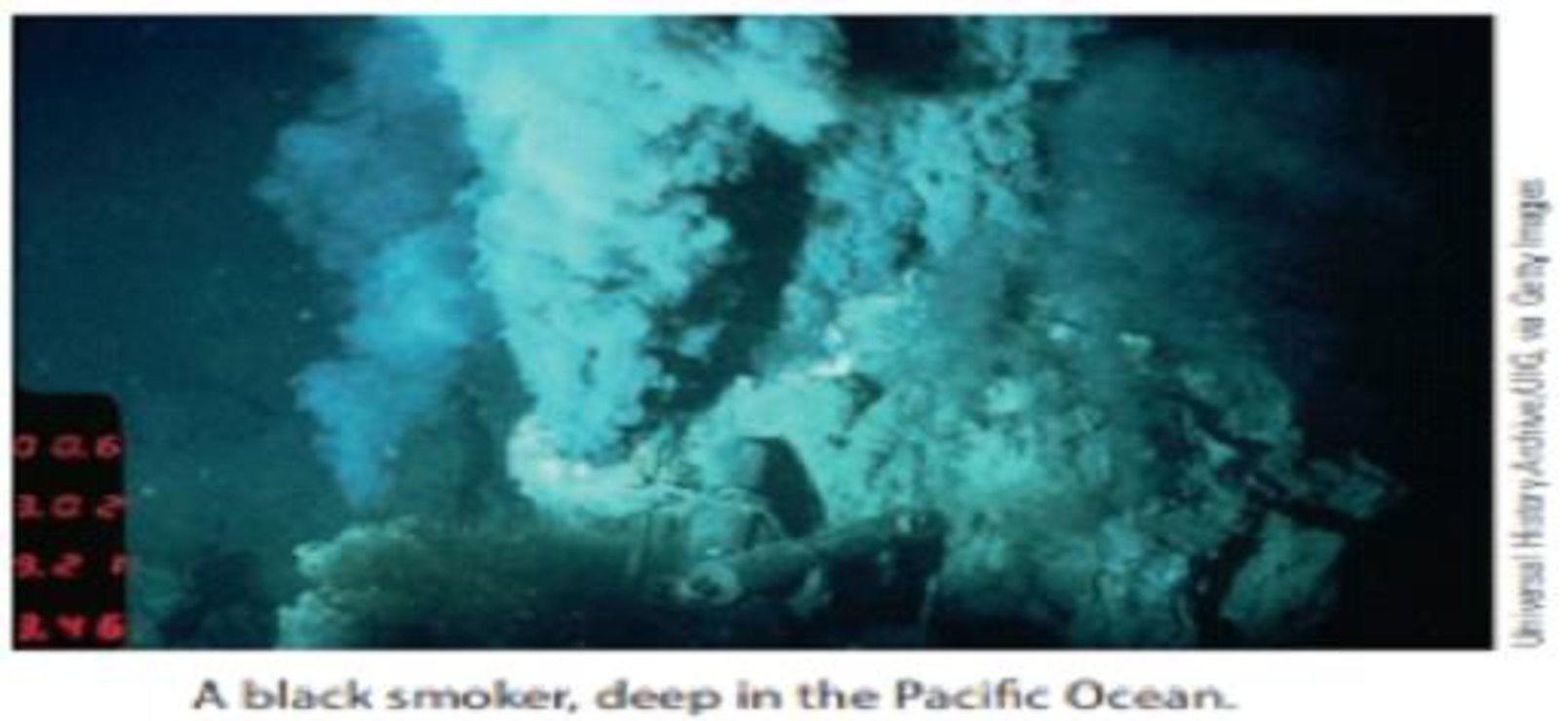Chapter 4, Problem 22PS

Chapter
Section
Textbook Problem

Black smokers are found in the depths of the oceans. Thinking that the conditions in these smokers might be conducive to the formation of organic compounds, two chemists in Germany found the following reaction could occur in similar conditions.2 CH3SH + CO → CH3COSCH3 + H2SIf you begin with 10.0 g of CH3SH and excess CO, (a) What is the theoretical yield of CH3COSCH3? (b) If 8.65 g of CH3COSCH3 is isolated, what is its percent yield?a)

Interpretation Introduction

Interpretation:

Theoretical yield and percent yield of CH3COSCH3 in the given condition has to be determined.

Concept introduction:

• The number of moles of  substance can be determined  using the equation,

Numberofmole=GivenmassofthesubstanceMolarmass

• The molar mass of an element or compound is the mass in grams of 1 mole of that substance, and it is expressed in the unit of grams per mol (g/mol).
• For chemical reaction balanced chemical reaction equation written in accordance with the Law of conservation of mass.
• Law of conservation of mass states that for a reaction total mass of the reactant and product must be equal.
• Theoretical yield: It is the maximum mass of product that can be obtained from a chemical reaction
• A measure of the extent of the reaction in terms of actual / the theoretical yield is referred as percentage yield.

Percentageyield=actualyieldtheoretical yield×100

Explanation

Balanced chemical equation for the given reaction is,

2CH3SH+COCH3COSCH3+H2S

To find the mass of product formed in the reaction, the amount of reactant CH3SH involved in the given reaction should be determined.

Therefore,

AmountofCH3SH=10gCH3SH×1molCH3SH48.11g=0.20785molCH3SH

The mass of CH3COSCH3 expected based on CH3SH reactant is,

0

b)

Interpretation Introduction

Interpretation:

Percent yield of CH3COSCH3 in the given condition has to be determined.

Concept introduction:

• The number of moles of  substance can be determined  using the equation,

Numberofmole=GivenmassofthesubstanceMolarmass

• The molar mass of an element or compound is the mass in grams of 1 mole of that substance, and it is expressed in the unit of grams per mol (g/mol).
• For chemical reaction balanced chemical reaction equation written in accordance with the Law of conservation of mass.
• Law of conservation of mass states that for a reaction total mass of the reactant and product must be equal.
• Theoretical yield: It is the maximum mass of product that can be obtained from a chemical reaction
• A measure of the extent of the reaction in terms of actual / the theoretical yield is referred as percentage yield.

Percentageyield=actualyieldtheoretical yield×100

Still sussing out bartleby?

Check out a sample textbook solution.

See a sample solution

The Solution to Your Study Problems

Bartleby provides explanations to thousands of textbook problems written by our experts, many with advanced degrees!

Get Started

Name the following compounds. a CaO b Mn2O3 c NH4HCO3 d Cu(NO3)2

General Chemistry - Standalone book (MindTap Course List)

5.34 Define the term mole fraction

Chemistry for Engineering Students

What is genomics?

Human Heredity: Principles and Issues (MindTap Course List)